SEARCH HOMEMath Central Quandaries & QueriesQuestion from Alise, a student: Hi Maths Central! I was wondering if you could help me with multiplying in base 5. I have read a question previously answered by you in regards to this but got fairly confused by it and was hoping you could help me with my question. My question is: Multiply 1422 base 5 by 21 base 5 keeping these numbers and answer in base 5. Would you also be able to explain how to get to the answer? Thanks!Hi Alise,

I'll do a similar problem for you.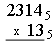Start to multiply as you would in base ten. 3 times 4 is twelve and twelve is $22_5$ so write 2 as the units digit in the first row of the multiplication and carry 2.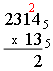Now multiply 3 times 1 to get 3 and add the carry of 2 to get five. But five in base five is $10_5$ so write a 0 in the first row of the multiplication and carry a 1.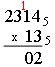Next multiply 3 times 3 to get nine and add 1 to get ten which is $20_5$ so write another 0 and a carry of 2.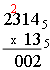To finish the first row of the multiplication multiply 3 times 2 to get six and add the carry of 2 to get eight which is $13_5$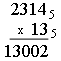Now move to the second row but this is easy since the multiplier is 1 so you get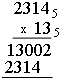All that remains is to add. The first three columns are easy and then in the fourth column 3 plus 3 is six which is $11_5$ so write a 1 and carry a 1 to get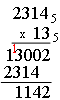Finally 2 plus 1 is 3 and adding the carry of 1 gives 4 so the result is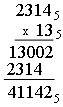Now try your problem and write back if you need more help.

PennyMath Central is supported by the University of Regina and the Imperial Oil Foundation.